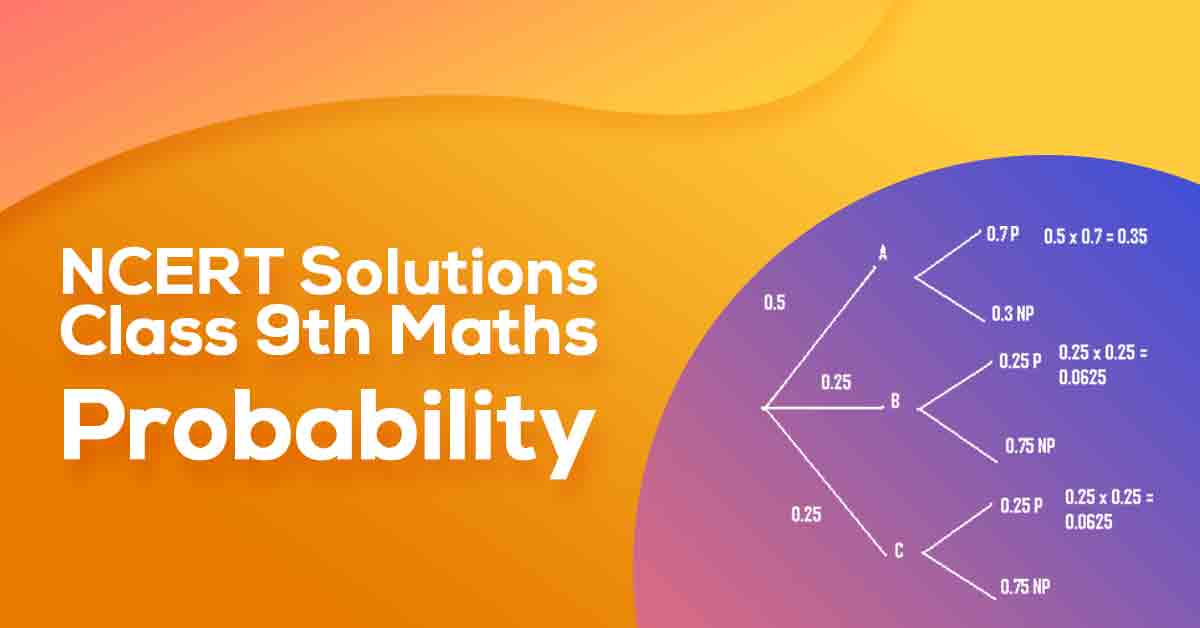Class 9th Math Probability NCERT Solution CBSE 2023

# NCERT Solutions For Class 9 Maths Chapter 15## NCERT Solutions For Class 9 Maths Chapter 15 Probability - PDF Download

Students can take help from NCERT Solutions for Class 9 Maths Chapter 15 Probability to study for their examinations. In this chapter, you will learn. An event for an experiment is the collection of some outcomes of the experiment. The empirical (or experimental) probability P(E) of an event E is given by P(E) = Number of trials in which E has happened/Total number of trials.

These CBSE NCERT Solutions for Class 9 Maths Chapter 15 Probability will help you to practice all the topics in the chapter with ease and score maximum marks in the examination.